# Rectangle - 7th grade (12y) - math problems

#### Number of problems found: 197

• AlexandraAlexandra made a rectangular quilt the measured 3 1/4. 2 3/4 feet in width. What is the area of the quilt in square feet? Write an equation to solve.
• Frame image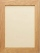Peter wants to put a picture in a frame. The picture is 7 3/5 cm wide. To fit in the picture frame cannot be more than 7 3/10 cm wide. How much should the image be trimmed?
• A rectangle 7A rectangle that has been split into 24 parts, set in 4 rows of 6 parts each. The parts in the top 3 rows have been shaded blue. The parts in the left 5 columns have been shaded purple. The first 5 parts in the top 3 rows are striped to show they are shad
• Matilda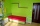Matilda measures the perimeter of her bedroom to determine how many feet of border she needs to go around the tops of the walls. Two walls are each 96 inches long, and two walls are each 3 yards long. She pays \$30 for border that costs \$1 per foot. What a
• Perimeter - rectangleFind the perimeter of the rectangle. A rectangle with a base of (3 5/6) m and a height of (2 3/7) m Its perimeter is x= meters. (Use fraction in your answer. )
• A rectangular 4A rectangular garden has a length of 57 m and a width of 42 m. Calculate of how many m2 will decrease the area of a garden, if the ornamental fence with a width of 60 cm will be planted inside its perimeter.
• Long and wideWhat is the area of a rectangle that is 1 1/4 meters long and 3/4​ of a meter wide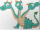Three sides of a quadrilateral are equal to the foreside which is 16 cm long. What is the length of the one with equal sides if the perimeter is 58 cm?
• A piece 3A piece of rectangle cloth has an area of 2 4/5 meters. Its breadth is 1 1/5 meters what is the length of the cloth?
• Picture 2Picture free is of length 14.2 cm and breadth 10.4 cm. The frame has border of uniforms with 2.6 cm. Find the area of photograph.
• The diagonals 2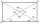The diagonals AC and BD of a rectangle ABCD intersect each other at a point O. If OA=4cm, find AC and BD.
• The length 5The length and breadth of rectangular field is 3 1/5 m  and 1 3/7 m. Find the area of rectangular field.
• A rectangular 3A rectangular plot of land has an area of 3/2 square kilometers and a length of ¾ kilometer.  What is the width of the plot of land?
• Mason 3Mason has a rectangular garden that is 2/3 yard wide by 7/4 yards long. What is the area of Mason’s garden? Use a drawing to show your work.
• The rugJosie has a rug with the area of 18 square feet. She will put the rug on the floor that is covered in 1/3 square foot tiles. How many tiles will the rug cover?
• Little 2Little fields farm lies on a rectangular plot of land that measures 2 2/4 miles in length and 2 2/3 miles in width. What is it's area in square miles?
• Marcos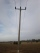Marcos wants to buy grass seed to cover his whole lawn, except for the pool. The pool is 7 1/2 m by 3 1/4 m. Find the area the grass seed needs to cover. Dimensions of the lawn are 50 m x 150 m.
• Sides of a rectangleThe dimensions of a rectangle are in a 4:12 ratio. If the shorter side length is 12 cm, what is the length of the longer side in centimetres?
• What is 8What is the perimeter of a rectangle with side lengths of ¾ inch and ⅔?
• Rectangle areaWhat is the area of a rectangle that has a length of 4 feet and a width of 4/10 feet?

Do you have an exciting math question or word problem that you can't solve? Ask a question or post a math problem, and we can try to solve it.

We will send a solution to your e-mail address. Solved examples are also published here. Please enter the e-mail correctly and check whether you don't have a full mailbox.

Rectangle Problems. Examples for 7th grade (seventh).